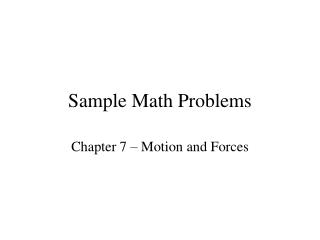Download PresentationSample Math Problems

Loading in 2 Seconds...

# Sample Math Problems - PowerPoint PPT Presentation

Sample Math Problems. Chapter 7 – Motion and Forces. An airplane traveling from San Francisco northeast to Chicago travels 1260 km in 3.5 hours. What is the airplane’s velocity?. v = d/t v = 1260 km 3.5 hours v = 360 km/h northeast. Question #15.I am the owner, or an agent authorized to act on behalf of the owner, of the copyrighted work described.
Download Presentation## Sample Math Problems

An Image/Link below is provided (as is) to download presentation

Download Policy: Content on the Website is provided to you AS IS for your information and personal use and may not be sold / licensed / shared on other websites without getting consent from its author.While downloading, if for some reason you are not able to download a presentation, the publisher may have deleted the file from their server.

- - - - - - - - - - - - - - - - - - - - - - - - - - E N D - - - - - - - - - - - - - - - - - - - - - - - - - -
Presentation Transcript
1. Sample Math Problems Chapter 7 – Motion and Forces

2. An airplane traveling from San Francisco northeast to Chicago travels 1260 km in 3.5 hours. What is the airplane’s velocity? v = d/t v = 1260 km 3.5 hours v = 360 km/h northeast Question #15

3. Heather and Matthew take 45 s to walk eastward along a straight road to a store 72 m away. What is their average velocity? v = d/t v = 72 m 45 s v = 1.6 m/s eastward Question #16

4. Simpson drives his car with an average velocity of 85 km/h toward the east. How long will it take him to drive 560 km on a perfectly straight highway? t = d/v t = 560 km 85 km/h t = 6.588235294 h t = 6.6 h Question #17

5. Calculate the momentum of an 85 kg man jogging north along the highway at 2.65 m/s. p = m*v p = (85 kg)(2.65 m/s) p = 225.25 kg*m/s p = 230 kg*m/s north Question #18

6. A driver is traveling east on a dirt road when she spots a pothole ahead. She slows her car from 14.0 m/s to 5.5 m/s in 6.0 s. What is the car’s acceleration? a = Δv/Δt a = (5.5 – 14.0 m/s) (6.0 - 0 s) a = -1.416666667 m/s2 a = -1.4 m/s2 east Question #20

7. A 5.5 kg watermelon is pushed across a table. If the acceleration of the watermelon is 4.2 m/s2 to the right, what is the net force acting on the watermelon? F = m*a F = (5.5 kg)(4.2 m/s2) F = 23.1 N F = 23 N Question #22

8. A block pushed with a force of 13.5 N accelerates at 6.5 m/s2 to the left. What is the mass of the block? m = F/a m = 13.5 N 6.5 m/s2 m = 2.076923077 kg m = 2.1 kg Question #23

9. The net force on a 925 kg car is 37 N as it pulls away from a stop sign. Find the car’s acceleration. a = F/m a = 37 N 925 kg a = 0.04 m/s2 a = 0.040 m/s2 a = 4.0 x 10-2 m/s2 away from the stop sign Question #24

10. A bag of sugar has a mass of 2.26 kg. What is its weight in newtons on the Earth? On Earth, g = 9.8 m/s2 w = m*g w = (2.26 kg)(9.8 m/s2) w = 22.148 N w = 22 N Question #25